# GRIM

library(scrutiny)

Granularity-related inconsistency of means, or GRIM, is a test for the mathematical consistency of reported means or proportions with the corresponding sample size (Brown and Heathers 2017). It can be applied to summary statistics of discrete numerical distributions. GRIM answers a simple question: Is it possible that a granular distribution has both the reported mean or percentage and the reported sample size?

This vignette covers scrutiny’s implementation of the GRIM test. It has the following sections — to get started, though, you only need the first one:

1. The basic grim() function and a specialized mapping function, grim_map().

2. Testing sequences of means or proportions.

3. The audit() method for summarizing grim_map()’s results.

4. The visualization function grim_plot().

5. Statistical benchmarks, such as granularity and the GRIM ratio.

## Basic GRIM testing

### Few cases: grim()

To test if a reported mean of 5.27 on a granular scale is GRIM-consistent with a sample size of 43, run this:

grim(x = "5.27", n = 43)
#>  5.27
#> FALSE

Note that x, the reported mean, needs to be a string. The reason is that strings preserve trailing zeros, which can be crucial for GRIM-testing. Numeric values don’t, and even converting them to strings won’t help. A workaround for larger numbers of such values, restore_zeros(), is discussed in vignette("wrangling").

grim() has some further parameters, but all of them can be used from within grim_map(). The other parameters will be discussed in that context because grim_map() is often the more useful function in practice. Furthermore, although grim() is vectorized, grim_map() is more safe and convenient for testing multiple combinations of means/proportions and sample sizes.

### Many cases: grim_map()

If you want to GRIM-test more than a handful of cases, the recommended way is to enter them into a data frame and to run grim_map() on the data frame. Two different ways to do that are discussed in vignette("wrangling"), but here, I will only describe an easily accessible solution for a single table.

Copy summary data from a PDF file and paste them into tibble::tribble(), which is available via scrutiny:

flying_pigs1 <- tribble(
~x,
"8.97",
"2.61",
"7.26",
"3.64",
"9.26",
"10.46",
"7.39"
) %>%
mutate(n = 28)

Use RStudio’s multiple cursors to draw quotation marks around all the x values, and to set commas at the end. See vignette("wrangling"), section With copy and paste, if you are not sure how to do that.

Now, simply run grim_map() on that data frame:

grim_map(flying_pigs1)
#> # A tibble: 7 × 4
#>   x         n consistency ratio
#>   <chr> <dbl> <lgl>       <dbl>
#> 1 8.97     28 FALSE        0.72
#> 2 2.61     28 TRUE         0.72
#> 3 7.26     28 FALSE        0.72
#> 4 3.64     28 TRUE         0.72
#> 5 9.26     28 FALSE        0.72
#> 6 10.46    28 TRUE         0.72
#> 7 7.39     28 TRUE         0.72

The x and n columns are the same as in the input. By default, the number of items composing the mean is assumed to be 1. The main result, consistency, is the GRIM consistency of the former three columns. On the ratio column, see section The GRIM ratio.

### Scale items

If a mean is composed of multiple items, set the items parameter to that number. Below are hypothetical means of a three-items scale. With the single-item default, half of these are wrongly flagged as inconsistent:

jpap_1 <- tribble(
~x,
"5.90",
"5.71",
"3.50",
"3.82",
"4.61",
"5.24",
) %>%
mutate(n = 40)

jpap_1 %>%
grim_map()  # default is wrong here!
#> # A tibble: 6 × 4
#>   x         n consistency ratio
#>   <chr> <dbl> <lgl>       <dbl>
#> 1 5.90     40 TRUE          0.6
#> 2 5.71     40 FALSE         0.6
#> 3 3.50     40 TRUE          0.6
#> 4 3.82     40 TRUE          0.6
#> 5 4.61     40 FALSE         0.6
#> 6 5.24     40 FALSE         0.6

Yet, all of them are consistent if the correct number of items is stated:

jpap_1 %>%
grim_map(items = 3)
#> # A tibble: 6 × 4
#>   x         n consistency ratio
#>   <chr> <dbl> <lgl>       <dbl>
#> 1 5.90    120 TRUE         -0.2
#> 2 5.71    120 TRUE         -0.2
#> 3 3.50    120 TRUE         -0.2
#> 4 3.82    120 TRUE         -0.2
#> 5 4.61    120 TRUE         -0.2
#> 6 5.24    120 TRUE         -0.2

It is also possible to include an items column in the data frame instead:

jpap_2 <- tribble(
~x,    ~items,
"6.92",  1,
"3.48",  1,
"1.59",  2,
"2.61",  2,
"4.04",  3,
"4.50",  3,
) %>%
mutate(n = 30)

jpap_2 %>%
grim_map()
#> # A tibble: 6 × 4
#>   x         n consistency ratio
#>   <chr> <dbl> <lgl>       <dbl>
#> 1 6.92     30 FALSE         0.7
#> 2 3.48     30 FALSE         0.7
#> 3 1.59     60 FALSE         0.4
#> 4 2.61     60 FALSE         0.4
#> 5 4.04     90 TRUE          0.1
#> 6 4.50     90 TRUE          0.1

### Percentage conversion

An underappreciated strength of GRIM is testing percentages. Since these are actually decimal numbers inflated by a factor of 100, percentages come with two “free” decimal places. However, percentages are often reported with decimal places beyond those two, which increases the probability of GRIM-inconsistencies unless true values were correctly reported.

Both grim() and grim_map() have a percent parameter which, if set to TRUE, divides the x values by 100 and increases the decimal count by two, so that percentages can be tested just like means:

jpap_3 <- tribble(
~x,     ~n,
"32.5",  438,
"35.6",  455,
"21.7",  501,
"39.3",  516,
)

jpap_3 %>%
grim_map(percent = TRUE)
#> ℹ x converted from percentage
#> # A tibble: 4 × 4
#>   x         n consistency ratio
#>   <chr> <dbl> <lgl>       <dbl>
#> 1 0.325   438 FALSE       0.562
#> 2 0.356   455 TRUE        0.545
#> 3 0.217   501 FALSE       0.499
#> 4 0.393   516 TRUE        0.484

### Reconstructed values

Set show_rec to TRUE if you want the values that were reconstructed during GRIM-testing to be displayed in the output. They will be columns prefixed with rec_:

pigs1 %>%
grim_map(show_rec = TRUE) %>%
dplyr::select(4:8)   # output cut down for printing
#> # A tibble: 12 × 5
#>    rec_sum rec_x_upper rec_x_lower rec_x_upper_rounded_up rec_x_upper_rounded_…¹
#>      <dbl>       <dbl>       <dbl>                  <dbl>                  <dbl>
#>  1  231.         7.25        7.22                    7.25                   7.25
#>  2  118.         4.76        4.72                    4.76                   4.76
#>  3  152.         5.24        5.21                    5.24                   5.24
#>  4   61.7        2.58        2.54                    2.58                   2.58
#>  5  183.         6.78        6.74                    6.78                   6.78
#>  6   75.0        2.71        2.68                    2.71                   2.71
#>  7  203.         7.03        7.00                    7.03                   7.03
#>  8  192.         7.38        7.35                    7.38                   7.38
#>  9   84.8        3.15        3.11                    3.15                   3.15
#> 10  214.         6.90        6.87                    6.9                    6.9
#> 11  125          5.00        5.00                    5                      5
#> 12    6.72       0.250       0.214                   0.25                   0.25
#> # … with abbreviated variable name ¹​rec_x_upper_rounded_down

• rec_sum: the sum total from which the mean or proportion was ostensibly derived.

• rec_x_upper: the upper reconstructed x value.

• rec_x_lower: the lower reconstructed x value.

• rec_x_upper_rounded_up: the rec_x_upper value rounded up.

• rec_x_upper_rounded_down: the rec_x_upper value rounded down.

• rec_x_lower_rounded_up: the rec_x_lower value rounded up.

• rec_x_lower_rounded_down: the rec_x_lower value rounded down.

The last four columns depend on rounding. Here, they follow the default "up_or_down", leading to two columns for each of rec_x_upper and rec_x_lower. With a singular rounding procedure, such as "up", there would only be one column each, and thus, two in total. The difference between these numbers is not greatly important, however, because rounding up and down mostly delivers the same results.

Internally, GRIM-consistency is determined by whether or not a stated x value is near-identical to either rec_x_upper_rounded or rec_x_lower_rounded. This algorithm follows the charitable and conservative protocol outlined by Brown and Heathers (2017). The rec_* columns were inspired by Bauer and Francis (2021)’s Table 1 but present values in slightly different ways.

### Rounding

The scrutiny package provides infrastructure for reconstructing rounded numbers. All of that can be commanded from within grim() and grim_map(). Several parameters allow for stating the precise way in which the original numbers have supposedly been rounded.

First and foremost is rounding. It takes a string with the rounding procedure’s name, which leads to the number being rounded in either of these ways:

1. Rounded "up" or "down" from 5. Note that SAS, SPSS, Stata, Matlab, and Excel round "up" from 5, whereas Python used to round "down" from 5.
2. Rounded to "even" using base R’s own round().
3. Rounded "up_from" or "down_from" some number, which then needs to be specified via the threshold parameter.
4. Given a "ceiling" or "floor" at the respective decimal place.
5. Rounded towards zero with "trunc" or away from zero with "anti_trunc".

The default, "up_or_down", allows for numbers rounded either "up" or "down" from 5 when GRIM-testing; and likewise for "up_from_or_down_from" and "ceiling_or_floor". For more about these procedures, see documentation for round(), round_up(), and round_ceiling(). These include all of the above ways of rounding.

Points 3 to 5 above list some quite obscure options that were only included to cover a wide spectrum of possible rounding procedures. The same is true for the threshold and symmetric parameters, so these aren’t discussed here any further. Learn more about scrutiny’s infrastructure for rounding at vignette("rounding").

By default, grim() and grim_map() accept values rounded either up or down from 5. If you have reason to impose stricter assumptions on the way x was rounded, specify rounding accordingly:

jpap_4 <- tibble::tribble(
~x,     ~n,
"2.02",  80,
"2.03",  80,
"2.04",  80,
"2.05",  80,
)

jpap_4 %>%
grim_map(rounding = "up")
#> # A tibble: 4 × 4
#>   x         n consistency ratio
#>   <chr> <dbl> <lgl>       <dbl>
#> 1 2.02     80 TRUE          0.2
#> 2 2.03     80 TRUE          0.2
#> 3 2.04     80 TRUE          0.2
#> 4 2.05     80 TRUE          0.2

jpap_4 %>%
grim_map(rounding = "down")
#> # A tibble: 4 × 4
#>   x         n consistency ratio
#>   <chr> <dbl> <lgl>       <dbl>
#> 1 2.02     80 TRUE          0.2
#> 2 2.03     80 TRUE          0.2
#> 3 2.04     80 TRUE          0.2
#> 4 2.05     80 TRUE          0.2

Rounding up and down leads to precisely opposite results for the first two cases, although it agrees on the latter two. Even this example is cherry-picked: Sample sizes of 80 are highly unusual because, for most other n values, those two rounding procedures agree in the vast majority of cases. See section Visualizing results with grim_plot() on this issue.

It might still be important to account for the different ways in which numbers can be rounded, if only to demonstrate that some given results are robust to those variable decisions. To err on the side of caution, the default for rounding is the permissive "up_or_down".

## Summarizing results with audit()

Following up on a call to grim_map(), the generic function audit() summarizes GRIM test results:

flying_pigs1 %>%
grim_map() %>%
audit() %>%
dplyr::select(1:5)   # output cut down for printing
#> # A tibble: 1 × 5
#>   incons_cases all_cases incons_rate mean_grim_ratio incons_to_ratio
#>          <int>     <int>       <dbl>           <dbl>           <dbl>
#> 1            3         7       0.429            0.72           0.595

These columns are —

1. incons_cases: number of GRIM-inconsistent value sets.

2. all_cases: total number of value sets.

3. incons_rate: proportion of GRIM-inconsistent value sets.

4. mean_grim_ratio: average of GRIM ratios.

5. incons_to_ratio: ratio of incons_rate to mean_ratio.

6. testable_cases: number of GRIM-testable value sets (i.e., those with a positive ratio).

7. testable_rate: proportion of GRIM-testable value sets.

## Visualizing results with grim_plot()

There is a specialized visualization function for GRIM test results, grim_plot():

jpap_5 <- tribble(
~x,        ~n,
"7.19",    28,
"4.56",    34,
"0.42",    27,
"1.31",    25,
"3.48",    34,
"4.27",    29,
"6.21",    30,
"3.11",    18,
"5.39",    36,
"5.66",    18,
)

jpap_5 %>%
grim_map() %>%
grim_plot()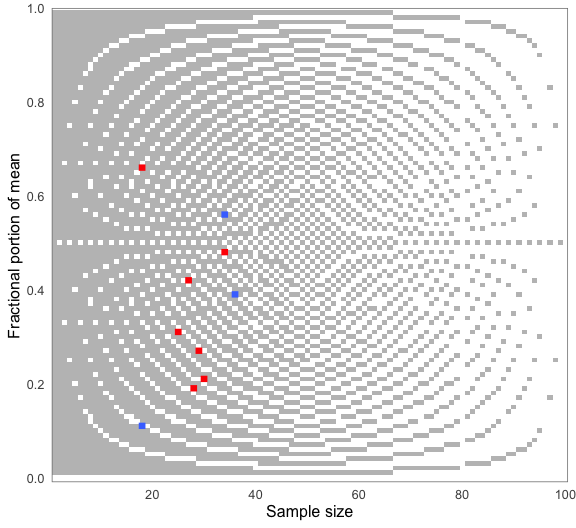grim_plot() can only be called on grim_map()’s output. It will fail otherwise:

grim_plot(mtcars)
#> Error in grim_plot():
#> ! grim_plot() needs GRIM or GRIMMER test results.
#> ✖ data is not grim_map() or grimmer_map() output.
#> ℹ The only exception is an "empty" plot that shows the background raster but no
#>   empirical test results. Create such a plot by setting show_data to FALSE.

With its unusual optics, this plot will probably not fit everyone’s taste. The results of grim_plot() are like those of error detection in general: They are not pretty, but they put the unvarnished truth on display.

The plot is strictly based on the laws governing GRIM. Its background raster shows all consistent (light) and inconsistent (dark) value pairs for two decimal places. Empirical values are shown in blue if consistent and red if inconsistent. Color settings and other ggplot2-typical options are available via arguments. Read about them at grim_plot()’s documentation.

You might notice the light vertical lines at $$N = 40$$ and $$N = 80$$: Few values are flagged as inconsistent here. This reflects grim_map()’s charitable default of accepting values rounded either up or down from 5. If a different rounding specification is chosen in the grim_map() call, the plot raster will adjust automatically (although it will often be the same as before):

jpap_5 %>%
grim_map(rounding = "ceiling") %>%
grim_plot()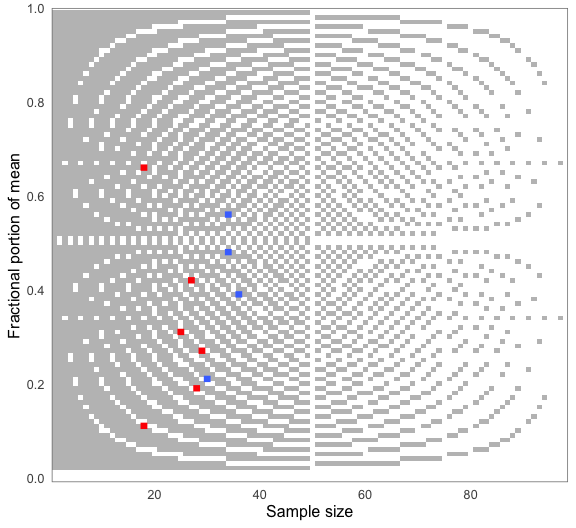All rounding values other than up_from, down_from, and up_from_or_down_from are supported.

Speed is not much of a concern here because all the rasters are based on data already stored within the package (in R/sysdata.rda), so they don’t need to be generated on the spot every time the function is called. See R/data-gen.R for the way they were generated.

## Testing numeric sequences with grim_map_seq()

GRIM analysts might be interested in a mean or percentage value’s numeric neighborhood. Suppose you found multiple GRIM inconsistencies as in out example pigs1 data. You might wonder whether they are due to small reporting or computing errors.

Use grim_map_seq() to GRIM-test the values surrounding the reported means and sample sizes:

out_seq1 <- grim_map_seq(pigs1)
out_seq1
#> # A tibble: 160 × 6
#>    x         n consistency ratio  case var
#>    <chr> <dbl> <lgl>       <dbl> <int> <chr>
#>  1 4.69     25 FALSE        0.75     1 x
#>  2 4.70     25 FALSE        0.75     1 x
#>  3 4.71     25 FALSE        0.75     1 x
#>  4 4.72     25 TRUE         0.75     1 x
#>  5 4.73     25 FALSE        0.75     1 x
#>  6 4.75     25 FALSE        0.75     1 x
#>  7 4.76     25 TRUE         0.75     1 x
#>  8 4.77     25 FALSE        0.75     1 x
#>  9 4.78     25 FALSE        0.75     1 x
#> 10 4.79     25 FALSE        0.75     1 x
#> # … with 150 more rows

### Summaries with audit_seq()

As this output is a little unwieldy, run audit_seq() on the results:

audit_seq(out_seq1)
#> # A tibble: 8 × 12
#>   x         n consistency hits_total hits_x hits_n diff_x diff_…¹ diff_…² diff_n
#>   <chr> <dbl> <lgl>            <int>  <int>  <int>  <dbl>   <dbl>   <dbl>  <dbl>
#> 1 4.74     25 FALSE                4      2      2      2       2      -2      2
#> 2 5.23     29 FALSE                6      3      3      1       1      -2      1
#> 3 2.57     24 FALSE                6      3      3      1       1      -3      1
#> 4 6.77     27 FALSE                7      3      4      1       1      -3      1
#> 5 7.01     29 FALSE                3      3      0      1       2      -1     NA
#> 6 3.14     27 FALSE                6      3      3      1       1      -3      1
#> 7 6.89     31 FALSE                8      4      4      1       1      -2      3
#> 8 0.24     28 FALSE                6      3      3      1       1      -3      1
#> # … with 2 more variables: diff_n_up <dbl>, diff_n_down <dbl>, and abbreviated
#> #   variable names ¹​diff_x_up, ²​diff_x_down

Here is what the output columns mean:

• x and n are the original inputs, reconstructed and tested for consistency here.

• hits is the number of GRIM-consistent value combinations found within the specified dispersion range.

• diff_x reports the absolute difference between x and the next consistent dispersed value (in dispersion steps, not the actual numeric difference). diff_x_up and diff_x_down report the difference to the next higher or lower consistent value, respectively.

• diff_n, diff_n_up, and diff_n_down do the same for n.

The default for dispersion is 1:5, for five steps up and down. When the dispersion sequence gets longer, the number of hits tends to increase:

out_seq2 <- grim_map_seq(pigs1, dispersion = 1:10)
audit_seq(out_seq2)
#> # A tibble: 8 × 12
#>   x         n consistency hits_total hits_x hits_n diff_x diff_…¹ diff_…² diff_n
#>   <chr> <dbl> <lgl>            <int>  <int>  <int>  <dbl>   <dbl>   <dbl>  <dbl>
#> 1 4.74     25 FALSE               12      6      6      2       2      -2      2
#> 2 5.23     29 FALSE               12      6      6      1       1      -2      1
#> 3 2.57     24 FALSE               11      6      5      1       1      -3      1
#> 4 6.77     27 FALSE               11      6      5      1       1      -3      1
#> 5 7.01     29 FALSE                6      6      0      1       2      -1     NA
#> 6 3.14     27 FALSE               13      6      7      1       1      -3      1
#> 7 6.89     31 FALSE               12      6      6      1       1      -2      3
#> 8 0.24     28 FALSE               13      6      7      1       1      -3      1
#> # … with 2 more variables: diff_n_up <dbl>, diff_n_down <dbl>, and abbreviated
#> #   variable names ¹​diff_x_up, ²​diff_x_down

### Visualizing GRIM-tested sequences

It’s curious what happens when we plot the output of grim_map_seq(). Like regular GRIM plots, however, it does give us a sense of how many tested values are consistent:

grim_plot(out_seq1)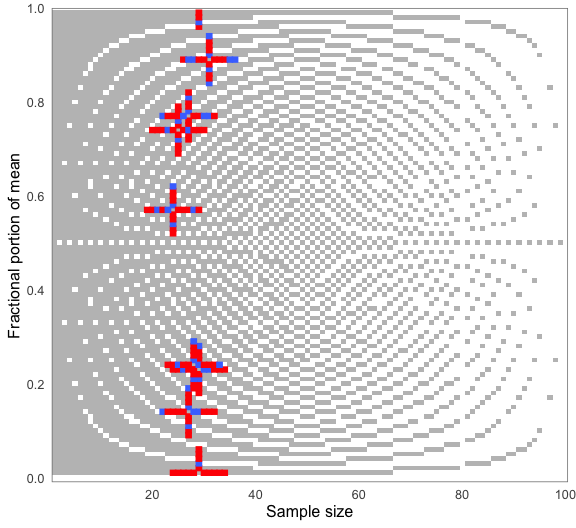The crosses appear because grim_map_seq() creates sequences around both x and n. Restrict this process to any one of these with the var argument:

out_seq1_only_x <- grim_map_seq(pigs1, var = "x")
out_seq1_only_n <- grim_map_seq(pigs1, var = "n")

grim_plot(out_seq1_only_x)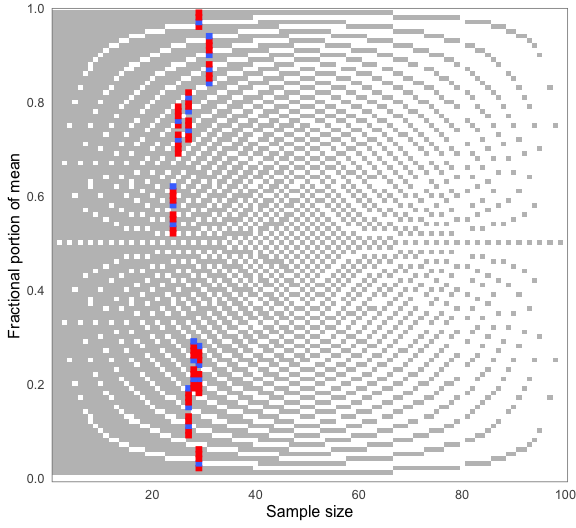grim_plot(out_seq1_only_n)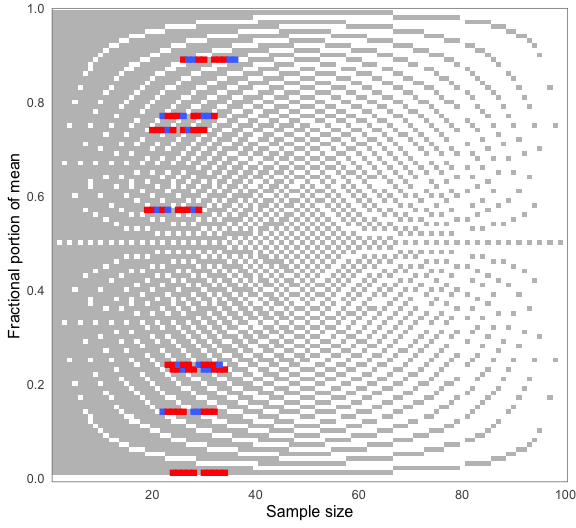## Handling unknown group sizes with grim_map_total_n()

### Problems from underreporting

Unfortunately, some studies that report group averages don’t report the corresponding group sizes — only a total sample size. This makes any direct GRIM-testing impossible because only x values are known, not n values. All that is feasible here in terms of GRIM is to take a number around half the total sample size, go up and down from it, and check which hypothetical group sizes are consistent with the reported group means. grim_map_total_n() semi-automates this process, motivated by a recent GRIM analysis (Bauer and Francis 2021).

The study examined by Bauer and Francis (2021) reported means of 5.3 and 4.71 for a total sample size of 40. With equal group sizes (i.e., 20 in each group), only 5.3 is GRIM-consistent, not 4.71. However, Bauer and Francis looked for a plausible scenario in which both means were consistent. They checked if those scenarios came about if the 40 participants were distributed across the two groups in slightly different ways.

More precisely, they went from a 20/20 group split to a 19/21 split, then to an 18/22 split, and finally to a 17/23 split. In the latter scenario, both means are consistent if 17 is paired with 4.71 and 23 with 5.3.

### Semi-automated solution

Instead of going about this manually, call grim_map_total_n(), followed by audit_total_n() for summarizing the results. It will find two further plausible scenarios in which both means are consistent; more on that below.

df <- tibble(x1 = "4.71", x2 = "5.3", n = 40)

# Detailed results:
df_tested <- grim_map_total_n(df)
df_tested
#> # A tibble: 24 × 8
#>    x         n n_change consistency both_consistent ratio  case dir
#>    <chr> <dbl>    <dbl> <lgl>       <lgl>           <dbl> <int> <chr>
#>  1 4.71     20        0 FALSE       FALSE            0.8      1 forth
#>  2 5.3      20        0 TRUE        FALSE           -1        1 forth
#>  3 4.71     19       -1 FALSE       FALSE            0.81     1 forth
#>  4 5.3      21        1 TRUE        FALSE           -1.1      1 forth
#>  5 4.71     18       -2 FALSE       FALSE            0.82     1 forth
#>  6 5.3      22        2 TRUE        FALSE           -1.2      1 forth
#>  7 4.71     17       -3 TRUE        TRUE             0.83     1 forth
#>  8 5.3      23        3 TRUE        TRUE            -1.3      1 forth
#>  9 4.71     16       -4 FALSE       FALSE            0.84     1 forth
#> 10 5.3      24        4 TRUE        FALSE           -1.4      1 forth
#> # … with 14 more rows

# Summary:
audit_total_n(df_tested)
#> # A tibble: 1 × 8
#>   x1    x2        n hits_total hits_forth hits_back scenarios_total hit_rate
#>   <chr> <chr> <dbl>      <dbl>      <dbl>     <dbl>           <dbl>    <dbl>
#> 1 4.71  5.3      40          3          1         2              12     0.25

Each “hit” is a scenario in which both x1 and x2 are GRIM-consistent with one of the two hypothetical group sizes. By default (dispersion = 0:5), the function goes five steps up and down from n.

### Testing both ways

Bauer and Francis (2021) only took those scenarios into account in which 4.71 was combined with the respective smaller group and 5.3 with the larger one, so 17/23 is the only “hit” they found (my term). However, the converse way of assigning hypothetical group sizes to the reported means would be equally justified. grim_map_total_n(), therefore, conducts two sets of GRIM tests: one for each way of pairing means and group sizes.

It thus finds the group sizes 19/21 and 16/24 to be GRIM-consistent if 5.3 is combined with the smaller group and 4.71 with the larger one (i.e., with pairing reversed from Bauer and Francis’ analysis). In the audit_total_n() summary of the function’s output, results from original pairing are named hits_forth, those from reversed pairing are named hits_back, and their sum is named hits_total.

This example only features one case — the df tibble has just a single row. It could have any number of rows, though; and grim_map_total_n() would determine and count the “hits” for each of them. See the Examples section of grim_map_total_n()’s documentation.

## GRIM statistics

### The GRIM ratio

#### Formula

The ratio column in a tibble returned by grim_map() is the “GRIM ratio”, i.e.:

$\frac{10^D - NL}{10^D}$

where $$D$$ is the number of decimal places in x (the mean or proportion), $$N$$ is the sample size, and $$L$$ is the number of scale items. Because $$N, L \geq 1$$, the GRIM ratio ranges from $$-\infty$$ to $$1 - \frac{1}{10^D}$$, asymptotically approaching 1. Its upper bound will be 0.9 if $$D = 1$$ and 0.99 if $$D = 2$$, etc.

#### Functions

grim_ratio() takes the arguments x, n, items, and percent as in grim() and grim_map():

grim_ratio(x = 1.42, n = 72)
#>  0.28

grim_ratio(x = 5.93, n = 80, items = 3)
#>  -1.4

# Enter x as a string to preserve trailing zeros:
grim_ratio(x = "84.20", n = 40, percent = TRUE)
#>  0.996

# Upper bounds:
grim_ratio_upper(x = 1.42)
#>  0.99
grim_ratio_upper(x = "84.20", percent = TRUE)
#>  0.9999

In addition, grim_total() takes the same arguments but returns only the numerator of the above formula:

grim_total(x = 1.42, n = 72)
#>  28

grim_total(x = 5.93, n = 80, items = 3)
#>  -140

grim_total(x = "84.20", n = 40, percent = TRUE)  # Enter x as string to preserve trailing zero
#>  9960

If grim_map()’s prob argument is set to TRUE, it adds a prob column that shows the probability of GRIM inconsistency. prob is derived from left-censoring the ratio column at 0, so it is equal to ratio if and only if $$0 \leq ratio$$. If $$ratio < 0$$, then $$prob = 0$$. (The GRIM ratio cannot be 1 or greater.)

#### Interpretation

If the GRIM ratio is non-negative, it can be interpreted as the proportion of inconsistent value sets corresponding to a given set of parameters. This is also the probability that a randomly chosen mean is GRIM-inconsistent. If the ratio is negative, the probability is 0.

Similarly, if the grim_total() value is non-negative, it can be interpreted as the total number of GRIM inconsistencies corresponding to a given set of parameters. If it is negative, that total is 0.

#### Origins

Although the term “GRIM ratio” is new, the formula is arguably implicit in Brown and Heathers’ (2017) paper on GRIM. The numerator is a transformation of the formula presented on p. 364, and the authors discuss a common special case of the ratio (interpreted as a proportion) on p. 367:

With reporting to two decimal places, for a sample size $$N < 100$$ [and a single item], a random mean value will be consistent in approximately $$N$$% of cases.

Assuming $$N = 70$$ and inserting all of these values into the above formula returns

$\frac{10^2-70×1}{10^2} = 0.3$

so that a random mean will be inconsistent in about 30% of cases and, conversely, consistent in about 70%.

Here is the same in code (assuming an arbitrary mean with two decimal places):

grim_ratio(x = 0.99, n = 70)
#>  0.3

Thus, all I did regarding the GRIM ratio was to make the general formula explicit and give it a name. Researchers may judge for themselves how useful it is for further analyses.

### Granularity and scale items

The granularity of a non-continuous distribution is the minimal amount by which two means or proportions of the distribution can differ. It is derived from the sample size and the number of scale items. The number of items, in turn, naturally follows from the distribution’s sample size and granularity.

#### Formulas

The granularity ($$G$$) formula is

$G = \frac{1}{NL}$

where $$N$$ is the sample size and $$L$$ is the number of items.

The scale items formula is the converse:

$L = \frac{1}{NG}$

#### Functions

Suppose you have an ordinal distribution with 80 observations and five items. To get its granularity, run this:

grim_granularity(n = 80, items = 4)
#>  0.003125

Now, imagine a distribution with 50 observations and a granularity of 0.01. To get the number of its items (actual or effective), use this code:

grim_items(n = 50, gran = 0.01)
#>  2

As the number of items itself has a granularity of 1, a call to grim_items() that doesn’t return whole numbers indicates a problem in earlier computations. A warning to that effect will be displayed:

grim_items(n = c(50, 65, 93), gran = 0.02)
#> Warning: 2 out of 3 item counts aren't whole numbers.
#> → This concerns 0.769 and 0.538.
#> ! Item counts have a granularity of 1, so they should be whole numbers. Are you
#>   sure about the n and gran values?
#>  1.0000000 0.7692308 0.5376344